$\newcommand{\NN}{\mathbb{N}} \newcommand{\CC}{\mathbb{C}} \newcommand{\GG}{\mathbb{G}} \newcommand{\LL}{\mathbb{L}} \newcommand{\PP}{\mathbb{P}} \newcommand{\QQ}{\mathbb{Q}} \newcommand{\RR}{\mathbb{R}} \newcommand{\VV}{\mathbb{V}} \newcommand{\ZZ}{\mathbb{Z}} \newcommand{\FF}{\mathbb{F}} \newcommand{\KK}{\mathbb{K}} \newcommand{\UU}{\mathbb{U}} \newcommand{\EE}{\mathbb{E}} \newcommand{\Aa}{\mathcal{A}} \newcommand{\Bb}{\mathcal{B}} \newcommand{\Cc}{\mathcal{C}} \newcommand{\Dd}{\mathcal{D}} \newcommand{\Ee}{\mathcal{E}} \newcommand{\Ff}{\mathcal{F}} \newcommand{\Gg}{\mathcal{G}} \newcommand{\Hh}{\mathcal{H}} \newcommand{\Ii}{\mathcal{I}} \newcommand{\Jj}{\mathcal{J}} \newcommand{\Kk}{\mathcal{K}} \newcommand{\Ll}{\mathcal{L}} \newcommand{\Mm}{\mathcal{M}} \newcommand{\Nn}{\mathcal{N}} \newcommand{\Oo}{\mathcal{O}} \newcommand{\Pp}{\mathcal{P}} \newcommand{\Qq}{\mathcal{Q}} \newcommand{\Rr}{\mathcal{R}} \newcommand{\Ss}{\mathcal{S}} \newcommand{\Tt}{\mathcal{T}} \newcommand{\Uu}{\mathcal{U}} \newcommand{\Vv}{\mathcal{V}} \newcommand{\Ww}{\mathcal{W}} \newcommand{\Xx}{\mathcal{X}} \newcommand{\Yy}{\mathcal{Y}} \newcommand{\Zz}{\mathcal{Z}} \newcommand{\al}{\alpha} \newcommand{\la}{\lambda} \newcommand{\ga}{\gamma} \newcommand{\Ga}{\Gamma} \newcommand{\La}{\Lambda} \newcommand{\Si}{\Sigma} \newcommand{\si}{\sigma} \newcommand{\be}{\beta} \newcommand{\de}{\delta} \newcommand{\De}{\Delta} \renewcommand{\phi}{\varphi} \renewcommand{\th}{\theta} \newcommand{\om}{\omega} \newcommand{\Om}{\Omega} \renewcommand{\epsilon}{\varepsilon} \newcommand{\Calpha}{\mathrm{C}^\al} \newcommand{\Cbeta}{\mathrm{C}^\be} \newcommand{\Cal}{\text{C}^\al} \newcommand{\Cdeux}{\text{C}^{2}} \newcommand{\Cun}{\text{C}^{1}} \newcommand{\Calt}{\text{C}^{#1}} \newcommand{\lun}{\ell^1} \newcommand{\ldeux}{\ell^2} \newcommand{\linf}{\ell^\infty} \newcommand{\ldeuxj}{{\ldeux_j}} \newcommand{\Lun}{\text{\upshape L}^1} \newcommand{\Ldeux}{\text{\upshape L}^2} \newcommand{\Lp}{\text{\upshape L}^p} \newcommand{\Lq}{\text{\upshape L}^q} \newcommand{\Linf}{\text{\upshape L}^\infty} \newcommand{\lzero}{\ell^0} \newcommand{\lp}{\ell^p} \renewcommand{\d}{\ins{d}} \newcommand{\Grad}{\text{Grad}} \newcommand{\grad}{\text{grad}} \renewcommand{\div}{\text{div}} \newcommand{\diag}{\text{diag}} \newcommand{\pd}{ \frac{ \partial #1}{\partial #2} } \newcommand{\pdd}{ \frac{ \partial^2 #1}{\partial #2^2} } \newcommand{\dotp}{\langle #1,\,#2\rangle} \newcommand{\norm}{|\!| #1 |\!|} \newcommand{\normi}{\norm{#1}_{\infty}} \newcommand{\normu}{\norm{#1}_{1}} \newcommand{\normz}{\norm{#1}_{0}} \newcommand{\abs}{\vert #1 \vert} \newcommand{\argmin}{\text{argmin}} \newcommand{\argmax}{\text{argmax}} \newcommand{\uargmin}{\underset{#1}{\argmin}\;} \newcommand{\uargmax}{\underset{#1}{\argmax}\;} \newcommand{\umin}{\underset{#1}{\min}\;} \newcommand{\umax}{\underset{#1}{\max}\;} \newcommand{\pa}{\left( #1 \right)} \newcommand{\choice}{ \left\{ \begin{array}{l} #1 \end{array} \right. } \newcommand{\enscond}{ \left\{ #1 \;:\; #2 \right\} } \newcommand{\qandq}{ \quad \text{and} \quad } \newcommand{\qqandqq}{ \qquad \text{and} \qquad } \newcommand{\qifq}{ \quad \text{if} \quad } \newcommand{\qqifqq}{ \qquad \text{if} \qquad } \newcommand{\qwhereq}{ \quad \text{where} \quad } \newcommand{\qqwhereqq}{ \qquad \text{where} \qquad } \newcommand{\qwithq}{ \quad \text{with} \quad } \newcommand{\qqwithqq}{ \qquad \text{with} \qquad } \newcommand{\qforq}{ \quad \text{for} \quad } \newcommand{\qqforqq}{ \qquad \text{for} \qquad } \newcommand{\qqsinceqq}{ \qquad \text{since} \qquad } \newcommand{\qsinceq}{ \quad \text{since} \quad } \newcommand{\qarrq}{\quad\Longrightarrow\quad} \newcommand{\qqarrqq}{\quad\Longrightarrow\quad} \newcommand{\qiffq}{\quad\Longleftrightarrow\quad} \newcommand{\qqiffqq}{\qquad\Longleftrightarrow\qquad} \newcommand{\qsubjq}{ \quad \text{subject to} \quad } \newcommand{\qqsubjqq}{ \qquad \text{subject to} \qquad } \newcommand{\eqdef}{\equiv}$

Semi-discrete Optimal Transport

# Semi-discrete Optimal Transport

This numerical tour studies semi-discrete optimal transport, i.e. when one of the two measure is discrete. It is not inteded to show efficient algorithm but only conveys the main underlying idea (c-transform, Laguerre cells, connexion to optimal quantization). In the Euclidean case, there exists efficient algorithm to compute Laguerre cells leveraging computational geometry algorithm for convex hulls.

## Dual OT and c-transforms

The primal Kantorovitch OT problem reads $W_c(\al,\be) = \umin{\pi} \enscond{\int_{\Xx \times \Yy} c(x,y) \text{d}\pi(x,y)}{ \pi_1=\al,\pi_2=\be }.$ It dual is $W_c(\al,\be) = \umax{f,g} \enscond{ \int_\Xx f \text{d} \al + \int_\Yy g \text{d} \be }{ f(x)+g(y) \leq c(x,y) }.$

We consider the case where $$\al=\sum_i a_i \de_{x_i}$$ is a discrete measure, so that the function $$f(x)$$ can be replaced by a vector $$(f_i)_{i=1}^n \in \RR^n$$. The optimal $$g(y)$$ function can the be replaced by the $$c$$-transform of $$f$$ $f^c(y) \eqdef \umin{i} c(x_i,y) - f_i.$

The function to maximize is then $W_c(\al,\be) = \umax{f \in \RR^n} \Ee(f) \eqdef \sum_i f_i a_i + \int f^c(y) \text{d}\be(y).$

We now implement a gradient ascent scheme for the maximization of $$\Ee$$. The evaluation of $$\Ee$$) can be computed via the introduction of the partition of the domain in Laguerre cells $\Yy = \bigcup_{i} L_i(f) \qwhereq L_i(f) \eqdef \enscond{y}{ \forall j, c(x_i,y) - f_i \leq c(x_j,y) - f_j }.$ Where $$f=0$$, this corrsponds to the partition in Voronoi cells.

One has that $$\forall y \in L_i(f)$$, $$f^c(y) = c(x_i,y) - f_i$$, i.e. $$f^c$$ is piecewise smooth according to this partition.

The grid for evaluation of the "continuous measure".

p = 500; % size of the image for sampling, m=p*p
t = linspace(0,1,p);
[V,U] = meshgrid(t,t);
Y = [U(:)';V(:)'];


First measure, sums of Dirac masses $$\al = \sum_{i=1}^n a_i \de_{x_i}$$.

n = 30;
X = .5+.5i + exp(1i*pi/4) * 1*( .1*(rand(1,n)-.5)+1i*(rand(1,n)-.5) );
X = [real(X);imag(X)];
a = ones(n,1)/n;


Second measure $$\be$$, potentially a continuous one (i.e. with a density), mixture of Gaussians. Here we discretize $$\beta = \sum_{j=1}^m b_j \de_{y_j}$$ on a very fine grid.

Z = {[.6 .9] [.4 .1]};  % mean
S = [.07 .09]; % variance
W = [.5 .5];
b = zeros(p); % ones(p)*.01;
for i=1:length(Z)
z = Z{i};
s = S(i);
b = b  + W(i) * exp( (-(U-z(1)).^2-(V-z(2)).^2)/(2*s^2) );
end
b = b/sum(b(:));


Display the two measures.

Col = rand(n,3);
clf; hold on;
imagesc(t,t,-b);
s = 60*ones(n,1); % size
scatter( X(2,:), X(1,:), s, .8*Col, 'filled' );
axis equal; axis([0 1 0 1]); axis off;
colormap gray(256);Initial potentials.

f = zeros(n,1);


compute Laguerre cells and c-transform

D = sum(Y.^2)' + sum(X.^2) - 2*Y'*X - f(:)';
[fC,I] = min(D,[], 2);
I = reshape(I, [p p]);


Dual value of the OT $$\dot{f}{a}+\dotp{f^c}{\be}$$.

OT = sum(f.*a) + sum(fC.*b(:));


Display the Laguerre call partition (here this is equal to the Vornoi diagram since $$f=0$$).

clf;
hold on;
imagesc(t,t,I); axis image; axis off;
contour(t,t,I, -.5:n+.5, 'k', 'LineWidth', 2);
colormap(Col);
scatter( X(2,:), X(1,:), (1+(f-min(f))*10)*100, Col*.8, 'filled' );Where $$\be$$ has a density with respect to Lebesgue measure, then $$\Ee$$ is smooth, and its gradient reads $\nabla \Ee(f)_i = a_i - \int_{L_i(f)} \text{d}\be(x).$

% sum area captured
r = sum(sum( ( I==reshape(1:n,[1 1 n]) ) .* b, 1 ),2); r = r(:);
nablaE = a-r(:);


Exercice 1: (check the solution) Implement a gradient ascent $f \leftarrow f + \tau \nabla \Ee(f).$ Experiment on the impact of $$\tau$$, display the evolution of the OT value $$\Ee$$ and of the Laguerre cells.

exo1;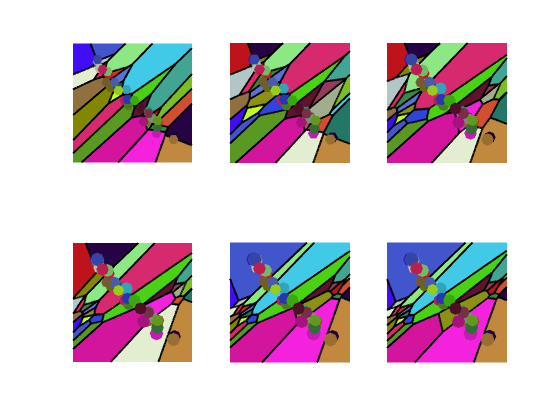Display the evolution of the estimated OT distance.

clf;
plot(1:niter, E, 'LineWidth', 2);
axis tight;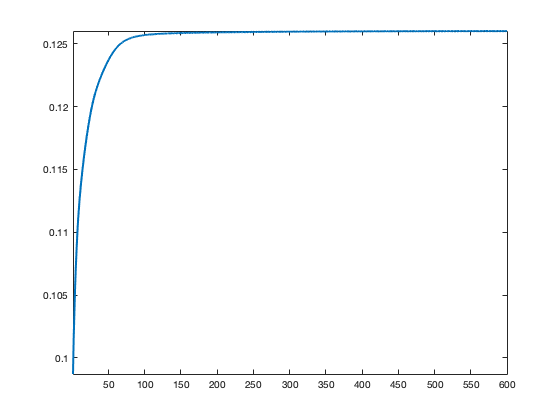## Stochastic Optimization

The function $$\Ee$$ to minimize can be written as an expectation over a random variable $$Y \sim \be$$ $\Ee(f)=\EE(E(f,Y)) \qwhereq E(f,y) = \dotp{f}{a} + f^c(y).$

One can thus use a stochastic gradient ascent scheme to minimize this function, at iteration $$\ell$$ $f \leftarrow f + \tau_\ell \nabla E(f,y_\ell)$ where $$y_\ell \sim Y$$ is a sample drawn according to $$\be$$ and the step size $$\tau_\ell \sim 1/\ell$$ should decay at a carefully chosen rate.

The gradient of the integrated functional reads $\nabla E(f,y)_i = a - 1_{L_i(f)}(y),$ where $$1_A$$ is the binary indicator function of a set $$A$$.

Initialize the algorithm.

f = 0;


Draw the sample.

i = (rand<W(1))+1; % select one of the two Gaussian
y = [S(i) * randn + Z{i}(1);S(i) * randn + Z{i}(2)];


% detect Laguerre cell where y is
[~,i] = min( sum(y.^2)' + sum(X.^2) - 2*y'*X - f(:)' );
nablaEy = a; nablaEy(i) = nablaEy(i) - 1;


Exercice 2: (check the solution) Implement the stochastic gradient descent. Test various step size selection rule.

exo2;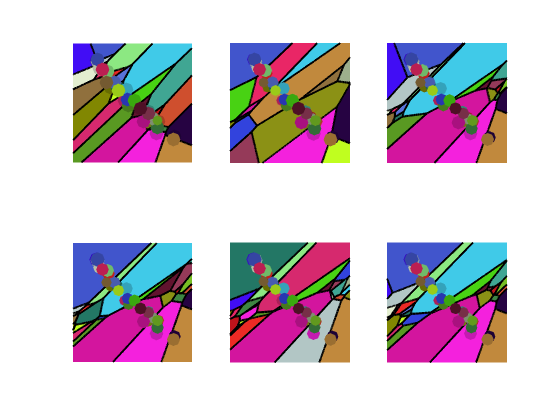Display the evolution of the estimated OT distance (warning: recording this takes lot of time).

clf;
plot(1:niter, E, 'LineWidth', 2);
axis tight;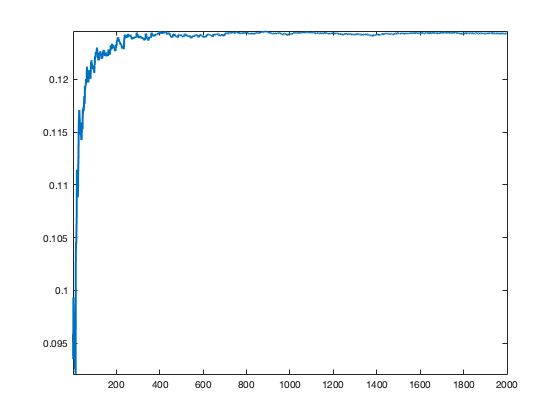## Optimal Quantization and Lloyd Algorithm

We consider the following optimal quantization problem $\umin{ (a_i)_i,(x_i)_i } W_c\pa{ \sum_i a_i \de_{x_i},\be }.$ This minimization is convex in $$a$$, and writing down the optimality condition, one has that the associated dual potential should be $$f=0$$, which means that the associated optimal Laguerre cells should be Voronoi cells $$L_i(0)=V_i(x)$$ associated to the sampling locations $V_i(x) = \enscond{y}{ \forall j, c(x_i,y) \leq c(x_j,y) }.$

The minimization is non-convex with respect to the positions $$x=(x_i)_i$$ and one needs to solve $\umin{x} \Ff(x) \eqdef \sum_{i=1}^n \int_{V_i(x)} c(x_i,y) \text{d} \be(y).$ For the sake of simplicity, we consider the case where $$c(x,y)=\frac{1}{2}\norm{x-y}^2$$.

The gradient reads $\nabla \Ff(x)_i = x_i \int_{V_i(x)} \text{d}\be - \int_{V_i(x)} y \text{d}\be(y).$ The usual algorithm to compute stationary point of this energy is Lloyd's algorithm, which iterate the fixed point $x_i \leftarrow \frac{ \int_{V_i(x)} y \text{d}\be(y) }{ \int_{V_i(x)} \text{d}\be },$ i.e. one replaces the centroids by the barycenter of the cells.

Intialize the centroids positions.

X1 = X;


Compute the Voronoi cells $$V_i(x)$$.

D = sum(Y.^2)' + sum(X1.^2) - 2*Y'*X1;
[fC,I] = min(D,[], 2);
I = reshape(I, [p p]);


Update the centroids to the barycenters.

A = ( I==reshape(1:n,[1 1 n]) ) .* b;
B = ( I==reshape(1:n,[1 1 n]) ) .* b .* ( U+1i*V );
X1 = sum(sum(B,1),2) ./ sum(sum(A,1),2);
X1 = [real(X1(:))';imag(X1(:))'];


Exercice 3: (check the solution) Implement Lloyd algortihm.

exo3;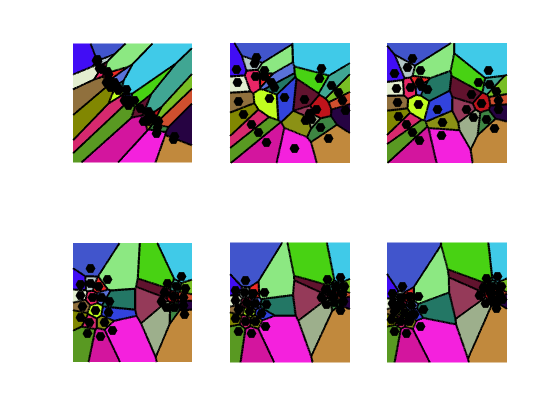Display the evolution of the estimated OT distance.

clf;
plot(1:niter, E, 'LineWidth', 2);
axis tight;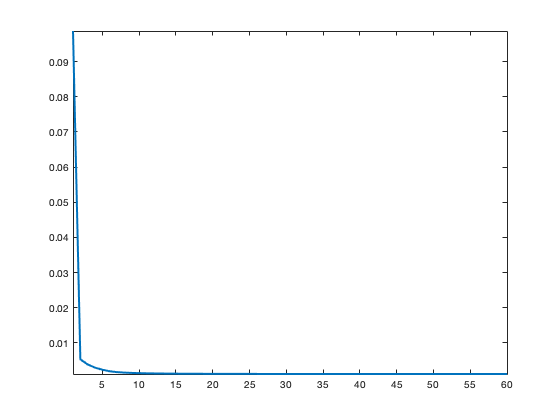## References

• Vladimir Oliker and Laird D Prussner. On the numerical solution of the equation $$\frac{\partial^2 z}{\partial x^2} \frac{\partial^2 z}{\partial y^2} - \pa{\frac{\partial^2 z}{\partial x\partial y}}^2 = f$$ and its discretizations, I. Numerische Mathematik, 54(3):271-293, 1989.
• Quentin Mérigot. A multiscale approach to optimal transport. Comput. Graph. Forum, 30(5):1583-1592, 2011.
• Bruno Lévy. A numerical algorithm for l2 semi-discrete optimal transport in 3d. ESAIM: Mathematical Modelling and Numerical Analysis, 49(6):1693-1715, 2015.
• Franz Aurenhammer, Friedrich Hoffmann, and Boris Aronov. Minkowski-type theorems and least-squares clustering. Algorithmica, 20(1):61-76, 1998.
• Franz Aurenhammer. Power diagrams: properties, algorithms and applications. SIAM Journal on Computing, 16(1):78-96, 1987.
• Guillermo Canas, Lorenzo Rosasco, L. Learning probability measures with respect to optimal transport metrics. In Advances in Neural Information Processing Systems, pp. 2492--2500, 2012.
• Peter M. Gruber. Optimum quantization and its applications. Adv. Math, 186:2004, 2002.
• Lloyd, Stuart P. (1982), "Least squares quantization in PCM", IEEE Transactions on Information Theory, 28 (2): 129?137,
• Aude Genevay, Marco Cuturi, Gabriel Peyré, and Francis Bach. Stochastic oppmization for large-scale optimal transport. In Advances in Neural Information Processing Systems, pages 3440-3448, 2016.